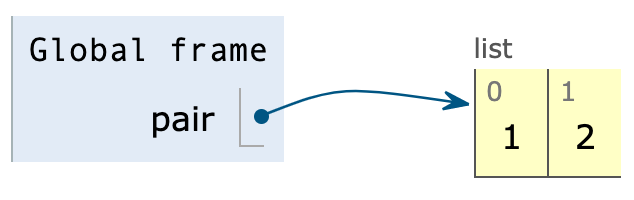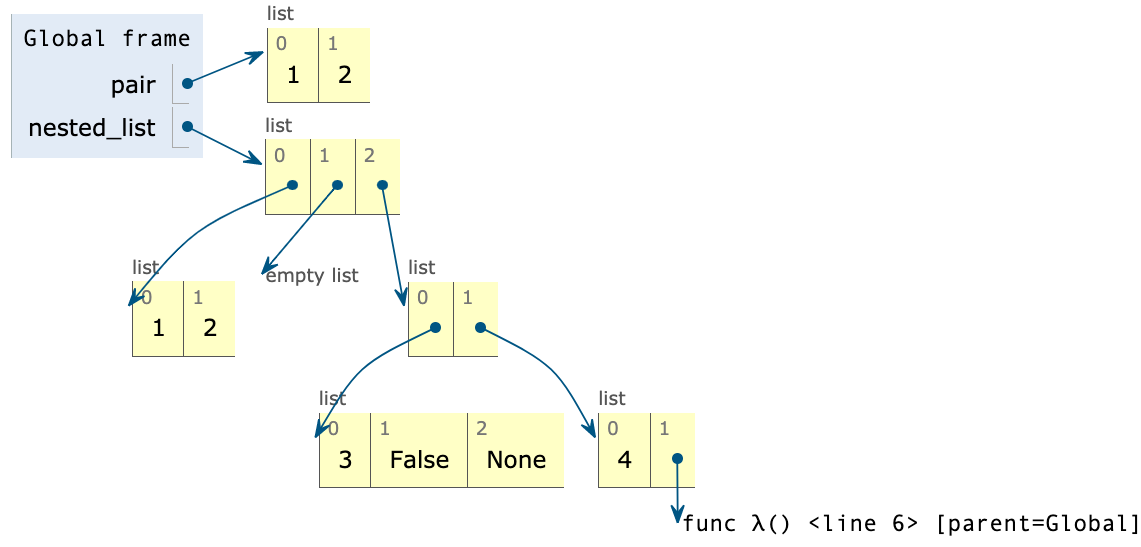# Sequences

Tips for navigating the slides:
• Press O or Escape for overview mode.
• Visit this link for a nice printable version
• Press the copy icon on the upper right of code blocks to copy the code

### Class outline:

• Box+Pointer
• Slicing
• Recursive exercises
• Built-ins for iterables

• Zoom chat: Good if you like getting responses from classmates or the lecture helper.
• Zoom Q&A: Good for asking questions that likely interest most students, and that should be answered in lecture.
• Post-lecture OH: Good for recapping a topic that went too fast. Or any questions!
• Piazza thread: Good for longer questions, tangential questions, or any unanswered questions.

## Box + Pointer

### Lists in environment diagrams

Lists are represented as a row of index-labeled adjacent boxes, one per element.

``````
pair = [1, 2]
``````### Nested lists in environment diagrams

Each box either contains a primitive value or points to a compound value.

``````
matrix = [ [1,2,0,4], [0,1,3,-1], [0,0,1,8] ]
``````### Nested lists in environment diagrams

A very nested list:

``````
worst_list = [ [1, 2],
[],
[ [3, False, None],
[4, lambda: 5]]]
``````## Slicing

### Slicing syntax

Slicing a list creates a new list with a subsequence of the original list.

``````
letters = ["A", "B", "C", "D", "E", "F"]
#   0    1    2    3    4    5

sublist1 = letters[1:]    # ['B', 'C', 'D', 'E', 'F']
sublist2 = letters[1:4]   # ['B', 'C', 'D']
``````

Slicing also works for strings.

``````
compound_word = "cortaúñas"

word1 = compound_word[:5]    # "corta"
word2 = compound_word[5:]   # "úñas"
``````

Negatives indices and steps can also be specified.

### Copying whole lists

Slicing a whole list copies a list:

``````
listA = [2, 3]
listB = listA

listC = listA[:]
listA = 4
listB = 5
``````

`list()` creates a new list containing existing elements from any iterable:

``````
listA = [2, 3]
listB = listA

listC = list(listA)
listA = 4
listB = 5
``````

Python3 provides more ways in the copy module.

## Recursion exercises

### Recursively sum a list

Let's code this up recursively:

``````
def sum_nums(nums):
"""Returns the sum of the numbers in NUMS.
>>> sum_nums([6, 24, 1984])
2014
>>> sum_nums([-32, 0, 32])
0
"""

``````

Docstrings typically would not specify whether an approach was recursive or iterative, since that is an implementation detail.

However, we'll make it clear in assignments and exam questions.

### Recursively sum a list (solution)

``````
def sum_nums(nums):
"""Returns the sum of the numbers in NUMS.
>>> sum_nums([6, 24, 1984])
2014
>>> sum_nums([-32, 0, 32])
0
"""
if (nums == []):
return 0
else:
return nums + sum_nums( nums[1:] )
``````

When recursively processing lists, the base case is often the empty list and the recursive case is often all-but-the-first items.

### Iteratively sum a range

Let's code this up iteratively:

``````
def sum_up_to(n):
"""Returns the sum of positive numbers from 1 up to N (inclusive).
>>> sum_up_to(5)
15
"""

``````

### Iteratively sum a range (solution)

Using the `range` type:

``````
def sum_up_to(n):
"""Returns the sum of positive numbers from 1 up to N (inclusive).
>>> sum_up_to(5)
15
"""
sum = 0
for n in range(0, n+1):
sum += n
return sum
``````

Remember that `range(start, end)` always ends right before `end`.

### Recursively sum a range

Now try it recursively:

``````
def sum_up_to(n):
"""Returns the sum of positive numbers from 1 up to N (inclusive).
>>> sum_up_to(5)
15
"""

``````

### Recursively sum a range (solution)

Now try it recursively:

``````
def sum_up_to(n):
"""Returns the sum of positive numbers from 1 up to N (inclusive).
>>> sum_up_to(5)
15
"""
if n == 1:
return 1
else:
return n + sum_up_to(n-1)
``````

## Reversing a string

### Recursively reversing a string

``````
def reverse(s):
"""Returns a string with the letters of S
in the inverse order.
>>> reverse('ward')
'draw'
"""
``````

Breaking it down into subproblems:

``````
reverse("ward") = reverse("ard") + "w"
reverse("ard") = reverse("rd") + "a"
reverse("rd") = reverse("d") + "r"
reverse("d") = "d"
``````

### Recursively reversing a string (solution)

``````
def reverse(s):
"""Returns a string with the letters of S
in the inverse order.
>>> reverse('ward')
'draw'
"""
if len(s) == 1:
return s
else:
return reverse(s[1:]) + s
``````

When recursively processing strings, the base case is typically an empty string or single-character string, and the recursive case is often all-but-the-first characters.

### Exercise: Reversing a number

``````
def reverse(n):
"""Returns N with the digits reversed.
>>> reverse_digits(123)
321
"""
``````

See walkthrough video here

### Helper functions

If a recursive function needs to keep track of more state than the arguments of the original function, you may need a helper function.

``````
def fUnKyCaSe(text):
"""Returns TEXT in fUnKyCaSe
>>> fUnKyCaSe("wats up")
'wAtS Up'
"""

def toggle_case(letter, should_up_case):
return letter.upper() if should_up_case else letter.lower()

def up_down(text, should_up_case):
if len(text) == 1:
else:

return up_down(text, False)

``````

### Recursion on different data types

Data type Base case condition Current item Recursive case argument
Numbers `== 0`
`== 1`
`n % 10` `n // 10`
Lists `== []` `L` `L[1:]`
`L[:-1]`
Strings `== ''`
`len(S) == 1`
`S` `S[1:]`
`S[:-1]`

## Built-in functions for iterables

### Functions that process iterables

The following built-in functions work for sequence types (lists, strings, etc) and any other iterable data type.

Function Description
`sum(iterable, start)` Returns the sum of values in `iterable`, initializing sum to `start`
`all(iterable)` Return `True` if all elements of `iterable` are true (or if `iterable` is empty)
`any(iterable)` Return `True` if any element of `iterable` is true. Return `False` if `iterable` is empty.
`max(iterable, key=None)` Return the max value in `iterable`
`min(iterable, key=None)` Return the min value in `iterable`

### Examples with sum/any/all

``````
sum([73, 89, 74, 95], 0)  # 331
``````
``````
all([True, True, True, True])     # True
any([False, False, False, True])  # True

all([x < 5 for x in range(5)])    # True

perfect_square = lambda x: x == round(x ** 0.5) ** 2
any([perfect_square(x) for x in range(50, 60)]) # False
``````

### Examples with max/min

``````
max([73, 89, 74, 95])         # 95
max(["C+", "B+", "C", "A"])   # C+
max(range(10))                # 9
``````

A key function can decide how to compare each value:

``````
coords = [ [37, -144], [-22, -115], [56, -163] ]
max(coords, key=lambda coord: coord)  # [56, -163]
min(coords, key=lambda coord: coord)  #  [-22, -115]
``````
``````
gymnasts = [ ["Brittany", 9.15, 9.4, 9.3, 9.2],
["Lea", 9, 8.8, 9.1, 9.5],
["Maya", 9.2, 8.7, 9.2, 8.8] ]
min(gymnasts, key=lambda scores: min(scores[1:]))    # ["Maya", ...]
max(gymnasts, key=lambda scores: sum(scores[1:], 0)) # ["Brittany", ...]
``````

## Python Project of The Day!

### Sea Level Rise

Sea Level Rise, by Douwe Osinga: Visualize sea levels and population density on interactive maps.Technologies used: Python (notebook) with PIL/numpy/Rasterio, HTML/CSS/JS with PanZoom
(Github repository)